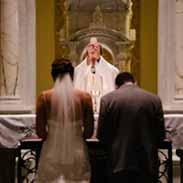# Quiz review for Obj. FRichard Lattimore
question

 carbon dioxide

 CO2
question

 CuS

 copper II sulfide
question

 magnesium nitride

 Mg3N2
question

 SrCl2

 strontium chloride
question

 P2O5

 diphosphorus pentaoxide
question

 BeI2

 beryllium iodide
question

 chromium IV sulfide

 CrS2
question

 SO2

 sulfur dioxide
question

 NaBr

 sodium bromide
question

 AsCl3

 arsenic trichloride
question

 Ni2O3

 nickel III oxide
question

 HgO

 mercury II oxide
question

 CaCl2

 calcium chloride
question

 BaS

 barium sulfide
question

 CdCl4
question

 MnO2

 manganese IV oxide
question

 Sb2O3

 antimony oxide
question

 SeCl2

 selenium dichloride
question

 CoCl2

 cobalt II chloride
question

 molybdenium III sulfide

 Mo2S3
question

 Ca3P2

 calcium phosphide
question

 AlP

 aluminum phosphide
question

 NO2

 nitrogen dioxide
question

 K2O

 potassium oxide
question

 copper I oxide

 Cu2O
question

 Iron III oxide

 Fe2O3
question

 ZnS

 zinc II sulfide
question

 NiI3

 nickel III iodide
question

 carbon disulfide

 CS2
question

 sulfur trioxide

 SO3
question

 KCl

 potassium chloride
question

 tungsten I carbide

 W4C
question

 SiO2

 silicon dioxide
question

 CrO2

 chromium IV oxide
question

 potassium sulfide

 K2S
question

 lithium sulfide

 Li2S
question

 CdO

question

 MgO

 magnesium oxide
question

 barium fluoride

 BaF2
question

 gallium arsenide

 GaAs
question

 Hg2S

 mercury I sulfide
question

 diphosphorus pentoxide

 P2O5
question

 aluminum bromide

 AlBr3
question

 diboron trioxide

 B2O3
question

 CrSe

 Chromium II selenide
question

 NiN

 nickel III nitride
question

 Al2O3

 aluminum oxide
question

 When non-metals combine with non-metals, both elements in the binary compound are trying to _____ electrons.

 GAIN
question

 Whenever Iron forms a compound with a non-metal, a ________ must appear in the name to indicate the valence of Iron.

 ROMAN NUMERAL
question

 Which family in the periodic table does not form binary compounds?

 GROUP 8A (the Nobel or Inert Gases)
question

 Metals will never form bonds with metals because both atoms are trying to _____ electrons.

 LOSE
question

 An atom that always forms a +3 ion would most likely be in which family?

 Group 3A
question

 An atom which forms a -3 ion would most likely be in which family?

 group 5A  (the Nitrogen family)
question

 When combined with the element sulfur, which one of the following elements would most likely come first in the formula? Br F Si O

 Si (because it is further to the left in the periodic table – more metallic – and would tend to lose electrons to the sulfur to become the (+) cation.
question

 ALL binary compounds end in the suffix _____.

 "ide"
question

 Which of the following elements would most likely form an anion? Fe Cl Ga Cs Mg

 Cl (it’s the only non-metal in the list;and would most likely gain electrons because it would;have the highest electronegativity value)
question

 CuBr

 copper I bromide
question

 V2S
question

 diphosphorus trisulfide

 P2S3
question

 MoS2

 Molybdinum IV sulfide
question

 Calculate the % of oxygen in the compound, dinitrogen tetraoxide.

 dinitrogen tetraoxide = N2O4; (F.W. = 92)64/92 X 100 = 69.6% oxygen
question

 Calculate the % of nitrogen in nitrogen triiodide.

 nitrogen;triiodide = NI3; (F.W. = 394.7)14/394.7 X 100 = 3.5 % nitrogen
question

 A compound is found to contain 70.0 % Iron and 30.0 % oxygen.; Determine the empirical formula from the above information and name the compound.

 Fe2O3Iron III oxide ; Step 1:; divide the percent by the element;F.W. from the periodic table Step 2:; divide the largest by the smallest numbers from the above step to get the empirical formula.
question

 How many grams are in 2.75 moles of Molybdinum sulfide?